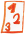Custom math worksheets at your fingertips# Details for problem "Insert relational operator - simple"

Quickname: 7029

## Summary

Insert the correct relational symbol between two numbers.

## Example## Description

Two natural numbers are given. The correct relational symbol has to be chosen and inserted in a blank space between the numbers.

The number of places for the natural numbers can be chosen. As an option, the number of places for the two number in a problem may be varied so that one of the numbers may have one place less than the other.

Download free worksheets for this math problem here. The worksheet contains the problems only, the solutions sheet includes the answers. Just click on the respective link.

•Worksheet 1Solution sheet with answers
•Worksheet 2Solution sheet with answers
•Worksheet 3Solution sheet with answers

If you can not see the solution sheets for download, they may be filtered out by an ad blocker that you may have installed. If this is the case, please allow ads for this page and reload the page. The solution sheets will then reappear.

• Do the sample worksheets do not really fit?
• Do you need more math worksheets, with a different level of difficulty?
• Would you like to combine different problems on a worksheet and adjust them to your needs?
• As a teacher, you can put together your own worksheets using the automatically generated math problems provided.
With a free initial credit, you can start creating your own math worksheets in a few minutes.

It does not cost anything to try! Register here, to create custom worksheets now!

## Customization options for this problem

Parameter
Possible values
Number of problems
1, 2, 3, 4, 5, 6, 7, 8, 9, 10
Places
1, 2, 3, 4, 5, 6
Vary no of places
Yes, No

## Similar problems

Remark
Description
similar problem, with fractions
Insert the correct relational symbol between two fractions
similar, with decimal fractions
Insert the correct relational symbol between two decimals.
compare product terms from the times table with numbers
Compare Times table multiplication terms to numbers, decide what is the correct relational symbol.

Deutsche Version dieser Aufgabe
These informational pages with samples describe math problems that can be combined on custom math worksheets with solutions for home and school use.